Learning Library

Subtraction Action: Beginning Math

These beginning math worksheets will get your kindergartener ready for elementary school arithmetic. The visual and numerical cues can help her use counting as an introduction to subtraction!

Click on a worksheet in the set below to see more info or download the PDF.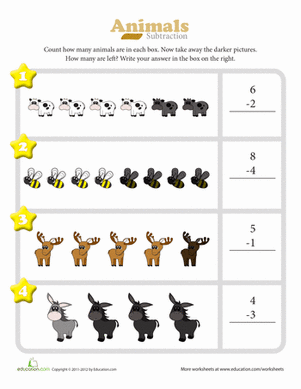Subtraction Action: Beginning Math

How many animals can you count? Get your kindergartener ready for elementary school math with this introduction to subtraction worksheet.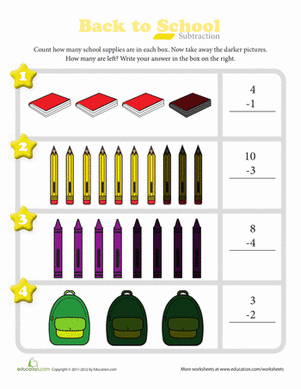Back to School Subtraction

How many back-to-school items can you count? Get your kindergartener ready for elementary school math with this introduction to subtraction worksheet.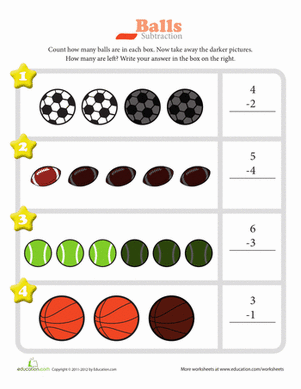Sports Star Subtraction

How many sports balls can you count? Get your kindergartener ready for elementary school math with this introduction to subtraction worksheet.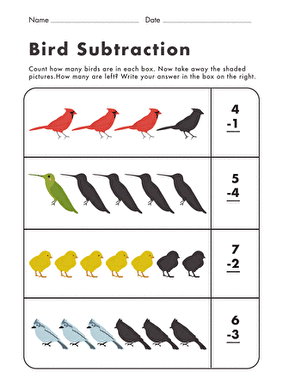Bird Subtraction

How many little birds can you count? Get your kindergartener ready for elementary school math with this introduction to subtraction worksheet.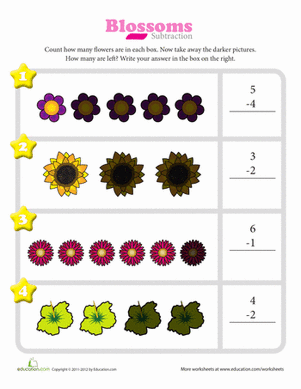Blossoms Subtraction

Visual and numerical cues serve as an introduction to subtraction as your kindergartener gets ready for elementary school arithmetic.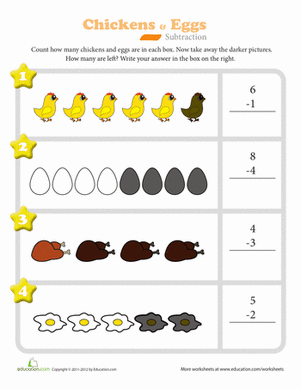Chicken or Egg Subtraction

How many chickens do you see? Get your kindergartener ready for elementary school math with this introduction to subtraction worksheet.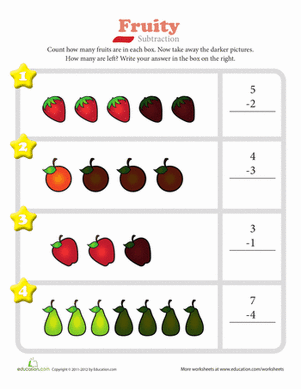Fruity Subtraction

How many delicious fruits can you count? Get your kindergartener ready for elementary school math with this introduction to subtraction worksheet.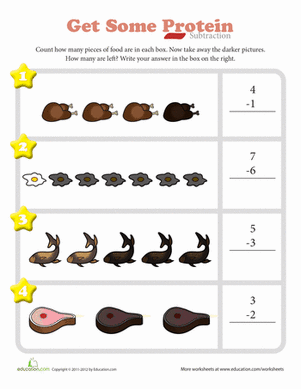Get Some Protein Subtraction

How many excellent sources of protein can you count? Get your kindergartener ready for elementary school math with this introduction to subtraction worksheet.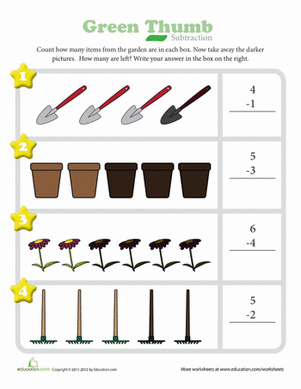Green Thumb Subtraction

How many gardening tools can you count? Get your kindergartener ready for elementary school math with this introduction to subtraction worksheet.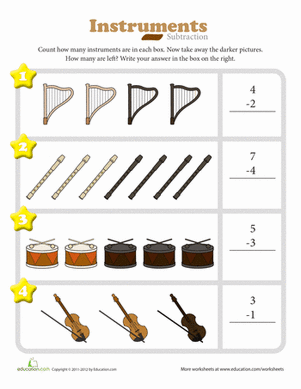Musical Instruments Subtraction

How many instruments can you count? Get your kindergartener ready for elementary school math with this introduction to subtraction worksheet.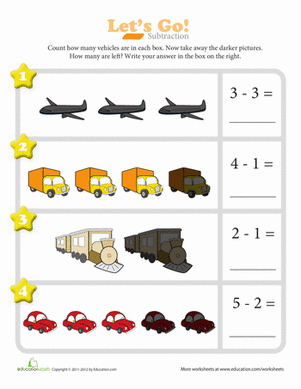Let's Go! Subtraction

Vroom vroom! How many vehicles can you count? Get your kindergartener ready for elementary school math with this introduction to subtraction worksheet.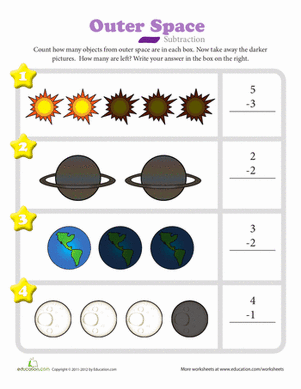Outer Space Subtraction

How many stars and planets can you count? Get your kindergartener ready for elementary school math with this introduction to subtraction worksheet.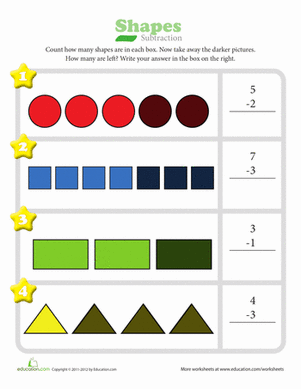Shapes Subtraction

How many circles and squares can you count? Get your kindergartener ready for elementary school math with this introduction to subtraction worksheet.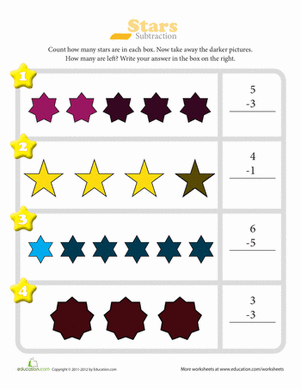Stars Subtraction

Don't get starry-eyed now! Count the stars and get your kindergartener ready for elementary school math with this introduction to subtraction worksheet.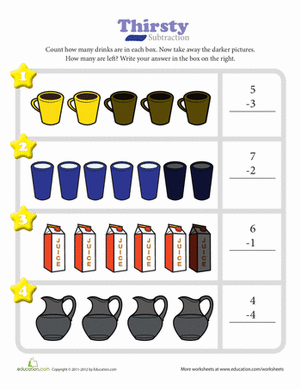Thirsty Subtraction

Thirsting for some math practice? Get your kindergartener ready for elementary school math with this introduction to subtraction worksheet.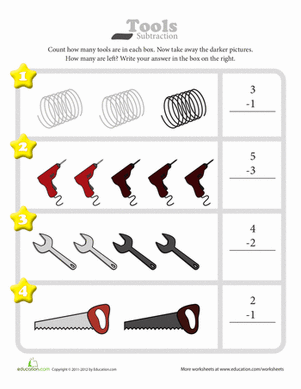Tools Subtraction

Put your little carpenter to work and count the tools! This beginning math worksheet will get your kindergartener ready for elementary school subtraction.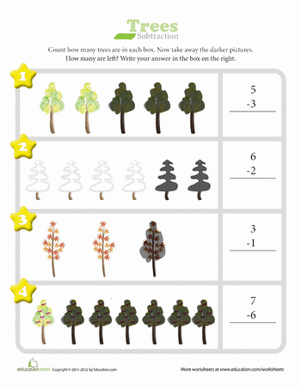Trees Subtraction

Hey treehuggers, how many trees can you count? Get your kindergartener ready for elementary school math with this introduction to subtraction worksheet.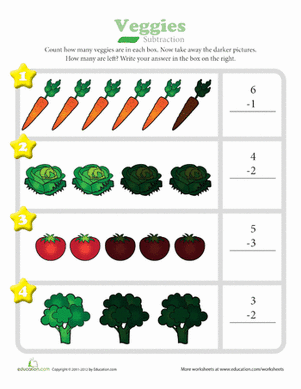Veggies Subtraction

How many veggies can you count? This beginning math worksheet will get your kindergartener ready for elementary school subtraction.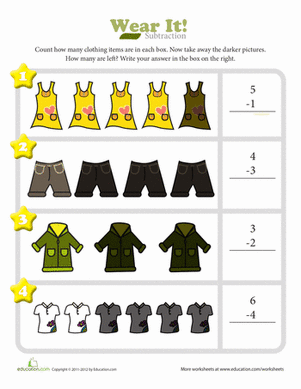Fashion Subtraction

How many clothes can you count? Get your kindergartener ready for elementary school math with this introduction to subtraction worksheet.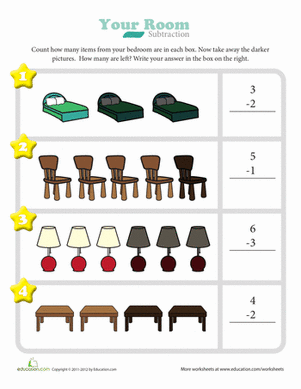Clean Your Room Subtraction

How many pieces of furniture can you count? Get your kindergartener ready for elementary school math with this introduction to subtraction worksheet.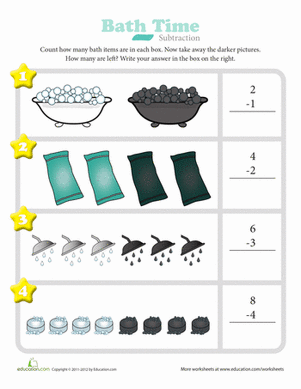Bath Time Subtraction

Introduce simple subtraction to your kindergartener. Count how many bath items there are in each box then take away the darker pictures to find the answer.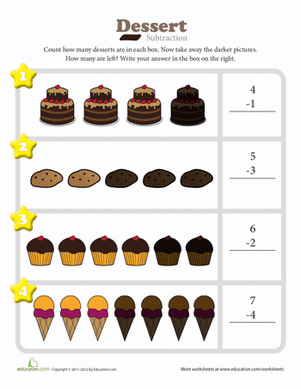Dessert Subtraction

Count the number of desserts in each box then take away the ones that are darker. How many are left?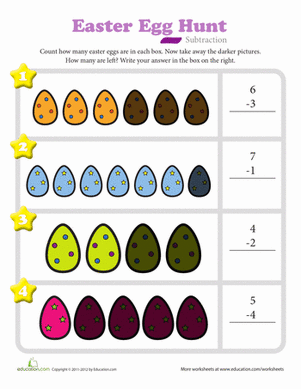Easter Egg Hunt Subtraction

Introduce your kindergartener to subtraction. Count the total number of eggs in each box. Then count the number of dark eggs and take that number away.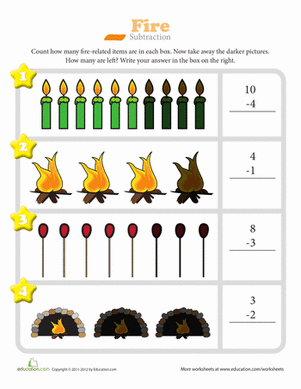Fire Subtraction

Kindergarteners will surely light up when completing this fun subtraction activity!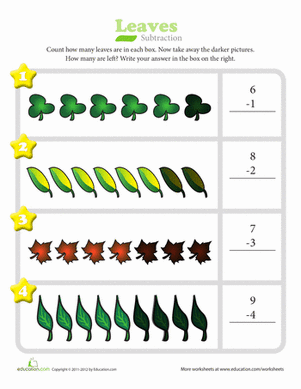Leaves Subtraction

Count the number of leaves in each box then take away the number of darker ones. Nurture the future math whiz in your child!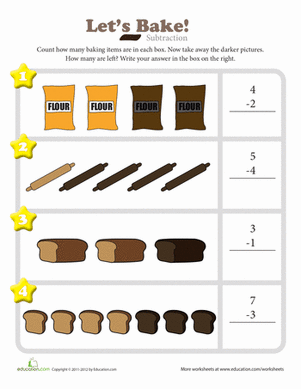Let's Bake! Subtraction

Practice subtraction by counting the number of baking items in each box then taking away the number of darker items. How many items are left?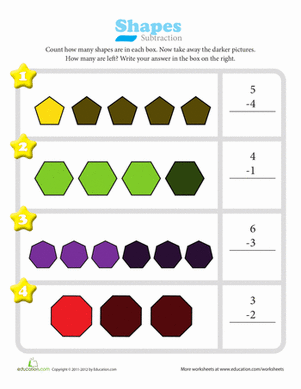Subtraction with Shapes

Practicing subtraction is simple. All you have to do is count the total number of shapes in each box then take away the darker shapes.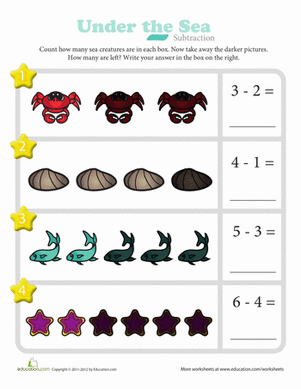Under the Sea Subtraction

Count the number of sea animals in each box then take away the number of darker ones to find the answers to the subtraction problems.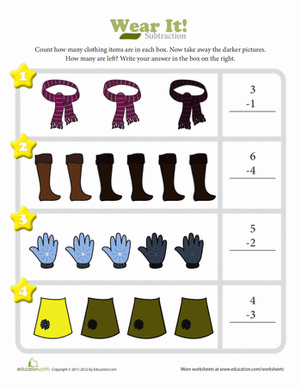Closet Subtraction

Count how many clothing items are in each box. Then take away the darker clothing items to find the answers to the simple subtraction problems.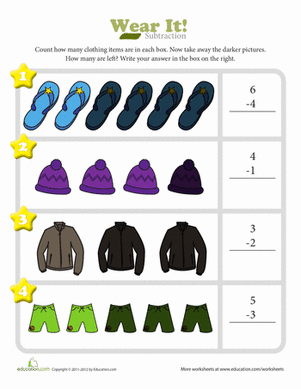Wear It! Subtraction

Practice simple subtraction by counting how many clothing items are in each box then take away the darker items.

Want to download this whole set as a single PDF?

Create new collection

0

New Collection>

0 items

What could we do to improve Education.com?

Please note: Use the Contact Us link at the bottom of our website for account-specific questions or issues.

What would make you love Education.com?